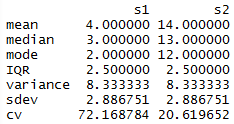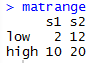Select Page

# A

Import vector of values of ice cream purchase numbers

>a <- c(8, 14, 16, 10, 11)

Generate random sample of 2 values and save to a vector

> b <- sample(a,2)
 10 11

Calculate mean and standard deviation of the sample.

>mean(b)
 10.5
> sd(b)
 0.7071068

Create data.frame out of mean and stdev for the sample and population.

mean(a)
 11.8
> sd(a)
 3.193744

smp <- c(10.5,0.7071068)
> pop <- c(11.8,3.193744)
> c <- data.frame < (smp, pop)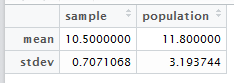# B

1. I think that the sample proportion will have the approximately the same distribution since nq = 5.
2. I think 100 is the smallest value of n for which p is approximately normal because anything smaller than n = 100 will make np < 5.  The high value of p  is very limiting.

## Bivariate Statistics

Initially, I was just going to calculate the correlation coefficient manually in R without using the functions, but then I decided that if I was going to do it manually then I may as well do it by hand.  I ended up making a mistake with spacing by not thinking about how many digits would be made out of squaring an already triple digit number.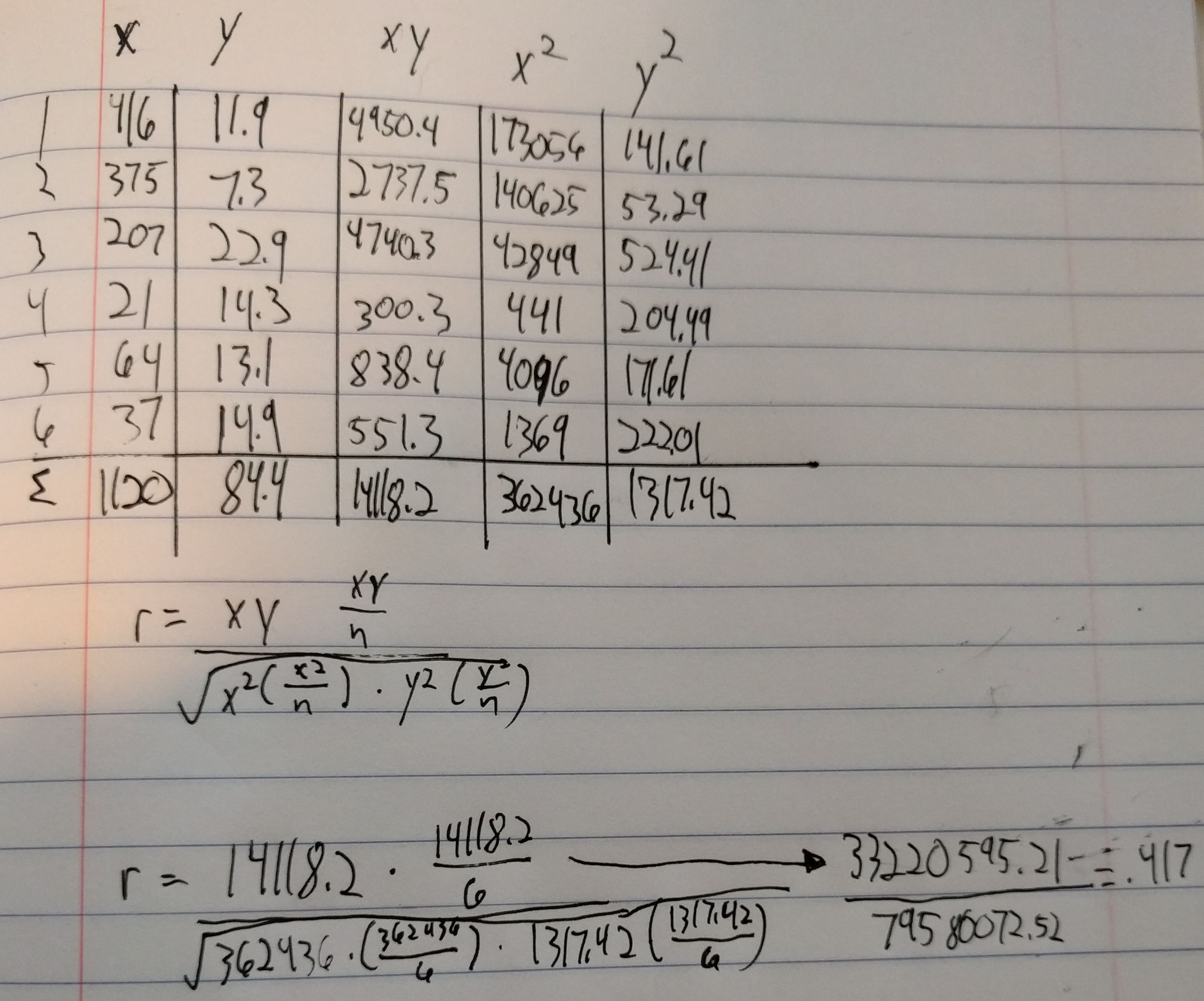From there, inputting the x and y values into R and executing the cor.tests() were very simple and straightforward.  I feel like I’ve gotten to the point with R where I don’t feel like I’m completely lost all the time.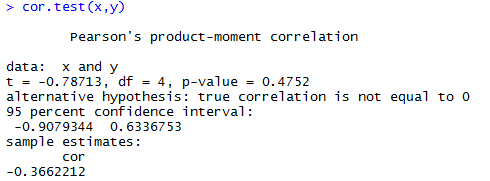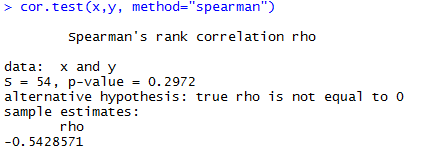## Module 2

For this assignment, I decided to to make everything as one script and use the Source with Echo command to run it all at once.  The only issue I had is that mode() does not yield a mode.  It returns what storage mode the object uses, so I had to look up how to make a function to calculate the mode.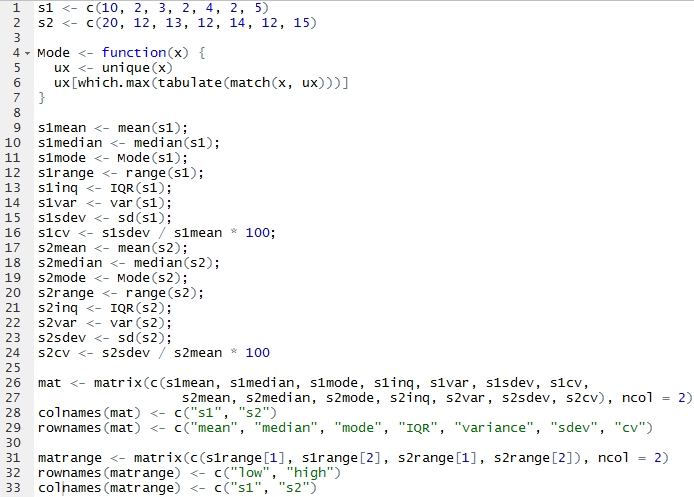From there, I was able to call mat and matrange to pull up the tables of all the values.